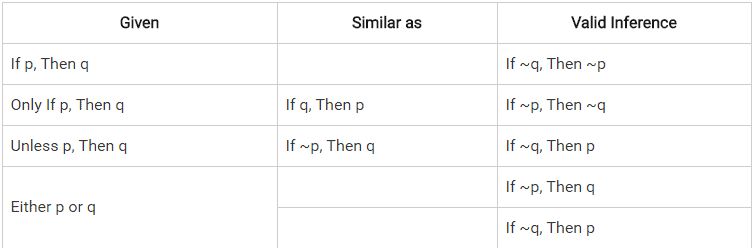Introduction & Examples (with Solutions): Logical Connectives

# Introduction & Examples (with Solutions): Logical Connectives - Notes | Study General Aptitude for GATE - Mechanical Engineering

 1 Crore+ students have signed up on EduRev. Have you?

Logical Connective (also called a logical operator) is a symbol or a word which is used to connect two or more sentences. Each logical connective can be expressed as a truth function.

### Logical connectives

1. NOT (Negation)
2. AND (Conjunction)
3. EITHER OR (Disjunction)
4. IF-THEN (Material Implication)

In logical reasoning, we deal with statements that are essentially sentences in English language. However, factual correctness is not important. We are only interested in logical truthfulness of the statements. We can represent simple statements using symbols like p and q. When simple statements are combines using logical connectives, compound statements are formed.

### Negation - NOT

Negation is the opposite of a statement. For example,

1. Statement: It is raining.
2. Negation: It is NOT raining.

### Disjunction - EITHER OR

When two statements are connected using OR, at least one of them is true. For example,

1. Either p or q: p alone is true; q alone is true; both are true

In such situation, valid inference is If p did not happen, then q must happen. And If p did not happen, then p must happen.

### Conjunction - AND

When two statements are connected using AND, both statements have to be true for compound statement to be true.

1. p and q: p should be true as well as q should be true

### Material Implication - IF THEN

If p, then q (p --> q): It is read as p implies q. It means that if we know p has occured, we can conclude that q has occured. In such situations, only valid inference is "If ~q, then ~p"; If q did not happen, then p did not happen.

### Negation of Compound Statements

1. Negation (p OR q) is same as Negation p AND Negation q
2. Negation (p AND q) is same as Negation p OR Negation q
3. Negation (p --> q) is same as Negation p --> Negation q

### Logical Connectives Summary TableExample

Examine the following statements:

1. I watch TV only if I am bored
2. I am never bored when I have my brother’s company.
3. Whenever I go to the theatre I take my brother along.

Which one of the following conclusions is valid in the context of the above statements?

1. If I am bored I watch TV
2. If I am bored, I seek my brother’s company.
3. If I am not with my brother, then I’ll watch TV.
4. If I am not bored I do not watch TV.

Ans.

1. Given = I watch TV only if I am bored
2. This is not in standard format. So first exchange position
3. Only if I’m bored (1), I watch TV(2)
4. What is the valid inference? Just look at the formula table
5. Only if 1=>2 then ~1=~2
6. Valid inference= if I’m not bored, I do not watch TV.
7. Look at the statements given in the answer choices, (D) matches. Therefore, final answer is (D).
The document Introduction & Examples (with Solutions): Logical Connectives - Notes | Study General Aptitude for GATE - Mechanical Engineering is a part of the Mechanical Engineering Course General Aptitude for GATE.
All you need of Mechanical Engineering at this link: Mechanical Engineering

## General Aptitude for GATE

73 videos|86 docs|108 tests
 Use Code STAYHOME200 and get INR 200 additional OFF

## General Aptitude for GATE

73 videos|86 docs|108 tests

Track your progress, build streaks, highlight & save important lessons and more!

,

,

,

,

,

,

,

,

,

,

,

,

,

,

,

,

,

,

,

,

,

;